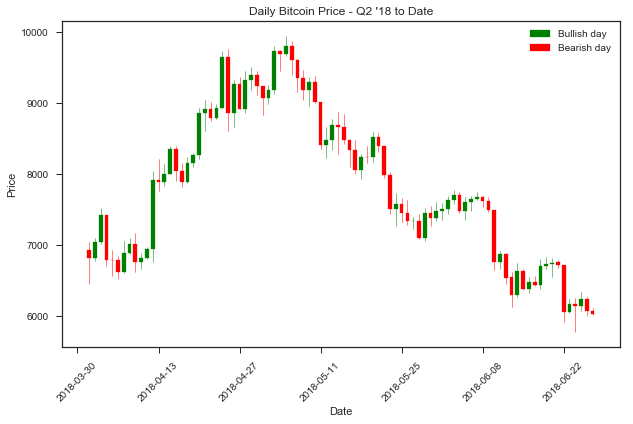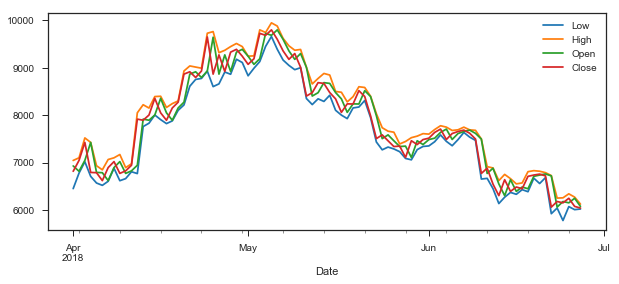This notebook explores visualizing historical price data for Bitcoin. A colorized candlestick chart helps visualize daily price trends and larger time frame price trends together: https://en.wikipedia.org/wiki/Candlestick_chart

## Candlestick Chart¶

Use matplotlib and mpl finance library for candlestick support: https://github.com/matplotlib/mpl_finance

Chart shows:

• Bitcoin prices organized by day (in UTC)
• X-axis is Date
• Y-axis is Price at Open, High, Low, and Close of the day

## Interpretation¶

• Color:
• Green candlesticks are "bullish" trend days (increased price)
• Red candlesticks are "bearish" trend days (decreased price)
• Body: Difference between open and close, day's trend strengh
• Shadows: Day's price range, high above open/close, low below open/close# Code¶

In :
import requests, json
import pandas as pd
import matplotlib.pyplot as plt
import matplotlib.dates as mdates
import matplotlib.patches as mpatches
from mpl_finance import candlestick_ohlc
from datetime import datetime

URL = 'https://api.gdax.com/'
COLS = ['Date', 'Low', 'High', 'Open', 'Close', 'Volume']
DAILY = 86400

def get_historical_prices(product):
r = requests.get(URL + f'/products/{product}/candles?granularity={DAILY}')
df = pd.DataFrame(r.json(), columns=COLS)
del df['Volume']
df['Date'] = pd.to_datetime(df.Date, unit='s')
df = df.set_index('Date')
return df.sort_index()

def plot_candlestick(df, ax=None, fmt="%Y-%m-%d", title=None):
if ax is None:
fig, ax = plt.subplots(label="Price")
idx_name = df.index.name
dat = df.reset_index()[[idx_name, "Open", "High", "Low", "Close"]]
dat[df.index.name] = dat[df.index.name].map(mdates.date2num)
ax.xaxis_date()
ax.xaxis.set_major_formatter(mdates.DateFormatter(fmt))
plt.xticks(rotation=45)
lines, patches = candlestick_ohlc(ax, dat.values, width=.65, colorup='g')
ax.set_xlabel("Date")
ax.set_ylabel("Price")
ax.set_title(title)
bullish = mpatches.Patch(color='green', label='Bullish day')
bearish = mpatches.Patch(color='red', label='Bearish day')
plt.legend(handles=[bullish, bearish])
return ax

bars = get_historical_prices('BTC-USD')['2018-04':]
bars.tail()

Out:
Low High Open Close
Date
2018-06-23 6040.01 6250.00 6059.82 6178.29
2018-06-24 5777.00 6255.80 6178.29 6149.99
2018-06-25 6070.13 6340.01 6150.00 6246.01
2018-06-26 6006.39 6271.00 6246.01 6074.95
2018-06-27 6020.00 6124.00 6074.01 6039.70

# Chart¶

In :
title = "Daily Bitcoin Price - Q2 '18 to Date"
fig, ax = plt.subplots(figsize=(10, 6))
ax = plot_candlestick(bars, ax=ax, title=title)### Comparison¶

Compare and contrast the above candlestick chart with the default line chart below.

Which is easier to intrepret day by day?

In :
bars.plot(figsize=(10, 4))

Out:
<matplotlib.axes._subplots.AxesSubplot at 0x1119edb38>In [ ]: